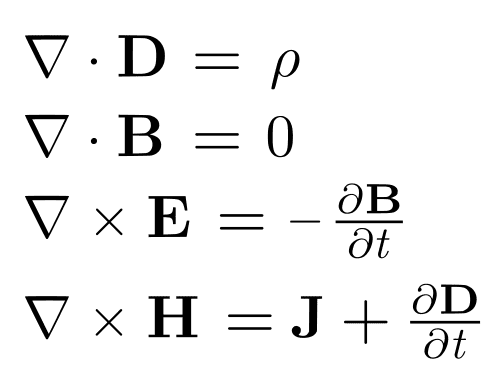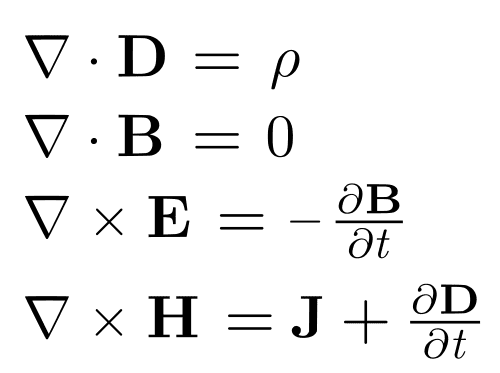# Over what frequency range are Maxwell's equations valid?

MathPi

## Homework Statement

I am studying for an Optics exam and in one of the practise tests is the following question: "Over what frequency range are Maxwell's equations valid?"

## Homework Equations

Maxwell's Equations

## The Attempt at a Solution

I've searched through my Griffiths Intro to electrodynamics, and all over Google, but came out short. Any leads would be appreciated.

Mentor

## Homework Statement

I am studying for an Optics exam and in one of the practise tests is the following question: "Over what frequency range are Maxwell's equations valid?"

## Homework Equations

Maxwell's Equations

## The Attempt at a Solution

I've searched through my Griffiths Intro to electrodynamics, and all over Google, but came out short. Any leads would be appreciated.
Do you see any frequency dependent terms in Maxwell's Equations? Are you familiar with how to work with differential equations like the linked system of Maxwell's Equations?MathPi

## Homework Statement

I am studying for an Optics exam and in one of the practise tests is the following question: "Over what frequency range are Maxwell's equations valid?"

## Homework Equations

Maxwell's Equations

## The Attempt at a Solution

I've searched through my Griffiths Intro to electrodynamics, and all over Google, but came out short. Any leads would be appreciated.

I should add that this is not homework for a class that I am taking, nor am I receiving any marks for answering this question.
Do you see any frequency dependent terms in Maxwell's Equations? Are you familiar with how to work with differential equations like the linked system of Maxwell's Equations?Yes I am familiar with solving DEs (I have a math degree). I am also familiar with Maxwells equations (I also have a physics degree). The question is literally as I have posted it - my best guess is that the these laws are not good at the quantum level and QED must be used (I am not too good at QED, I only took one course in subatomic physics).

I should add that this is not a homework question for a course I am taking. Nor am I receiving any marks for answering this.

Mentor
"Over what frequency range are Maxwell's equations valid?"
my best guess is that the these laws are not good at the quantum level and QED must be used (I am not too good at QED, I only took one course in subatomic physics).

MathPi
It's just a guess - trying to come to an answer through discussion. Any constructive input is appreciated :)

Mentor
I think you are over-thinking it.
Do you see any frequency dependent terms in Maxwell's Equations?
What level is this question anyway. From your OP it sounded like it was at a pretty basic level:
I am studying for an Optics exam
Opticians don't do much with QM.

OTOH, if this is for a Quantum Optics class, there may be a different answer, I suppose.

MathPi
I think you are over-thinking it.

What level is this question anyway. From your OP it sounded like it was at a pretty basic level:

Opticians don't do much with QM.

OTOH, if this is for a Quantum Optics class, there may be a different answer, I suppose.
Ah I should have specified the level. The exam is for an undergraduate optics at an advanced EM level (maybe 4th year physics).

MathPi
Ah I should have specified the level. The exam is for an undergraduate optics at an advanced EM level (maybe 4th year physics).

Even if I am overthinking it there are only two possibilities: either Maxwells equations are valid for all frequencies, or they are not. If not, what are the ranges and why?

Mentor
So QED may be involved? i'm not familiar with any frequency limitations of Maxwell's Equations, but are they modified for QED? That's kind of a different question.

MathPi
So QED may be involved? i'm not familiar with any frequency limitations of Maxwell's Equations, but are they modified for QED? That's kind of a different question.
I doubt QED is involved - that's too advanced for this course. Like I said it was just a guess - maybe not a good one! I cannot think of anything else besides that (trying to remember my subatomic physics course).

Mentor
At least from my perspective, frequency limitations do not come into effect with the basic Maxwell Equations. Probably a trick question...

•MathPi
MathPi
At least from my perspective, frequency limitations do not come into effect with the basic Maxwell Equations. Probably a trick question...
Yes you might be right. Perhaps I was hoping there was more to it. I'll take it as it is for now unless someone else has any thoughts. Thanks for your input!

•berkeman